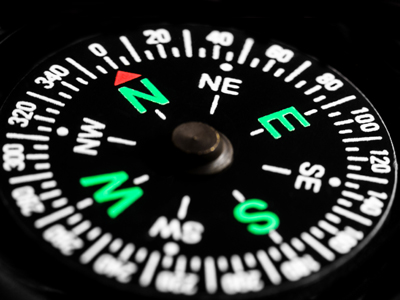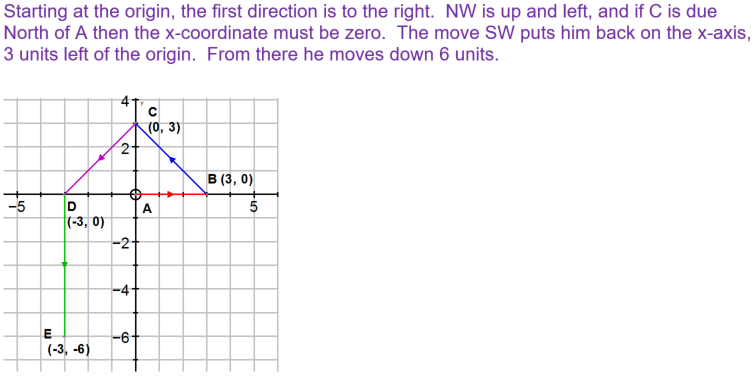Knowing the compass points will help when playing this quiz.

# Position 4

This Math quiz is called 'Position 4' and it has been written by teachers to help you if you are studying the subject at middle school. Playing educational quizzes is a fabulous way to learn if you are in the 6th, 7th or 8th grade - aged 11 to 14.

It costs only \$12.50 per month to play this quiz and over 3,500 others that help you with your school work. You can subscribe on the page at Join Us

In this middle school Math quiz on position you'll get a chance to see what you remember about the coordinate plane and the compass. Did you know that the coordinate plane is also called the Cartesian plane?

Did you know that map directions are given using bearings? Some people use a 'thumb compass' when reading maps. These are very basic compasses that normally only show magnetic north. They are used in orienteering. But if a compass is held over a map, how can people read what is underneath the map? Can you figure it out? It's easy really - the compasses are transparent so that the map-readers can see right through!

1.
Which direction does the straight line starting at (0, 0) and ending at (6, 6) point in?
NW
SE
NE
E
A diagram will help a lot in doing these questions
2.
What are the coordinates of the point that is the same distance away from the x-axis as the point
(0, 5)?
(0, -5)
(-5, 0)
(5, 0)
(5, 5)
(0, 5) is at 5 on the positive y-axis: 5 units above the x-axis. (0, -5) is at -5 on the negative y-axis: 5 below the x-axis
3.
If you join up the points A (0, 0), B (1, 0), C (1, -1), D (0, -1) with straight lines you will get a square that lies in a certain quadrant. If the square is rotated counterclockwise through 90° about the origin (0, 0), which quadrant will it end up in and what will its new coordinates be?
A (0, 0), B (1, 0), C (1, -1), D (0, 1) in the first quadrant
A (0, 0), B (0, 1), C (1, 1), D (1, 0) in the first quadrant
A (0, 0), B (0, -1), C (-1, -1), D (-1, 0) in the third quadrant
A (0, 0), B (1, 0), C (0, -1), D (-1, -1) in the third quadrant
The square is initially in the fourth quadrant. Join up the four corners with straight lines (the lengths of which should be 1 unit) to the center of rotation (0, 0). Now rotate the lines and watch where their end points end up: these will give you the positions of the new coordinates of the square. Draw it!
4.
Which coordinates are NW of (3, -3)?
(3, 3)
(-3, 3)
(-3, -3)
(3, -3)
(3, -3) is SE of (0, 0). Join up (0, 0) and (3, -3) with a straight line and rotate the line about (0, 0) through 180° to get to NW: you should end up at (-3, 3)
5.
Eddie is tracing out a straight line path in the Cartesian plane. He starts at A (0, 0) and moves as follows: 3 cm E to point B, then NW to point C, which is due North of point A. From there he moves the same distance SW to point D, and finally 6 cm S to point E, where he stops. What are the coordinates of point E?
E (-3, 6)
E (3, -6)
E (-3, -6)
E (3, 6)6.
You are facing NW. If you turn through 315° counterclockwise and then 225° clockwise, which direction will you be facing now?
W
E
NE
SW
315° counterclockwise = seven lots of 45° (seven-eighths of a turn) makes you face N. 225° clockwise = 5 lots of 45° = (five-eighths of a turn) makes you face SW
7.
You are facing NE. If you turn through 180° counterclockwise and then 135° clockwise, which direction will you be facing now?
N
NW
NE
E
'Counterclockwise' means that you turn (rotate) in the opposite direction to which the hands of the clock turn: the hands of the clock turn 'clockwise'. 180° = two lots of 90° (a half turn) counterclockwise makes you face SW. 135° clockwise = 3 lots of 45° = three-eighths of a turn makes you face N
8.
How many complete rotations are there in 3,249°?
9
7
12
8
There are 3,249° ÷ 360° = 9 complete rotations
9.
Which of the following is the angle between NE and S?
315°
45°
225°
135°
Three lots of 45°. If you rotated in the counterclockwise direction, it would be five lots of 45° = 225°. Whenever you can, always give the smallest angle. Note: 225° counterclockwise + 135° clockwise = 360°
10.
A circle with center (0, 0) and diameter 6 cm is drawn on the coordinate plane. Which points will the circle pass through?
(3, 0), (0, 3), (-3, 0), (0, -3)
(6, 0), (0, 6), (-6, 0), (0, -6)
(6, 0), (6, 6), (-6, 0), (0, -6)
(6, 0), (0, 6), (-6, -6), (0, -6)
The radius is half the diameter = 3 cm; therefore, the circle will pass through those points that are at a distance of 3 cm from its center (0, 0)
Author:  Frank Evans